##### Physics I For Dummies, 3rd EditionPicture a small child on a spinning playground ride, such as a merry-go-round, and she’s yelling that she wants to get off. You have to stop the spinning ride, but it’s going to take some effort. Why? Because it has angular momentum.

In physics, you can calculate angular momentum in the same way that you calculate linear momentum — just substitute moment of inertia for mass, and angular velocity for velocity.

## What is angular momentum?

Angular momentum is the quantity of rotation of a body, which is the product of its moment of inertia and its angular velocity.

Linear momentum, p, is defined as the product of mass and velocity:

p = mv

This is a quantity that is conserved when there are no external forces acting. The more massive and faster moving an object, the greater the magnitude of momentum.

## The angular momentum equation

Physics also features angular momentum, L. The equation for angular momentum looks like this: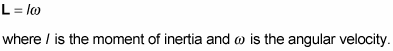The angular momentum equation features three variables:

• L = angular momentum
• / = the moment of inertia
• W = the angular velocity
Note that angular momentum is a vector quantity, meaning it has a magnitude and a direction.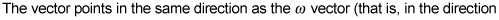the thumb of your right hand points when you wrap your fingers around in the direction the object is turning).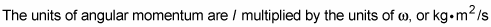in the MKS (meter-kilogram-second) system.

The important idea about angular momentum, much as with linear momentum, is that it’s conserved.

The principle of conservation of angular momentum states that angular momentum is conserved if no net torques are involved.

This principle comes in handy in all sorts of problems, such as when two ice skaters start off holding each other close while spinning but then end up at arm’s length. Given their initial angular velocity, you can find their final angular velocity, because angular momentum is conserved: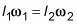If you can find the initial moment of inertia and the final moment of inertia, you’re set. But you also come across less obvious cases where the principle of conservation of angular momentum helps out. For example, satellites don’t have to travel in circular orbits; they can travel in ellipses. And when they do, the math can get a lot more complicated. Lucky for you, the principle of conservation of angular momentum can make the problems simple.

## Angular momentum example problem

Say that NASA planned to put a satellite into a circular orbit around Pluto for studies, but the situation got a little out of hand and the satellite ended up with an elliptical orbit. At its nearest point to Pluto,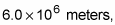the satellite zips along at 9,000 meters per second.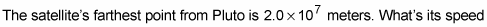at that point? The answer is tough to figure out unless you can come up with an angle here, and that angle is angular momentum.

Angular momentum is conserved because there are no external torques the satellite must deal with (gravity always acts parallel to the orbital radius). Because angular momentum is conserved, you can say thatBecause the satellite is so small compared to the radius of its orbit at any location, you can consider the satellite a point mass. Therefore, the moment of inertia, I, equals mr2. The magnitude of the angular velocity equals v/r, so you can express the conservation of angular momentum in terms of the velocity like so: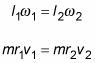You can put v2 on one side of the equation by dividing by mr2: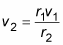You have your solution; no fancy math involved at all, because you can rely on the principle of conservation of angular momentum to do the work for you. All you need to do is plug in the numbers: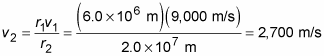At its closest point to Pluto, the satellite will be screaming along at 9,000 meters per second, and at its farthest point, it will be moving at 2,700 meters per second. Easy enough to figure out, as long as you have the principle of conservation of angular momentum under your belt.